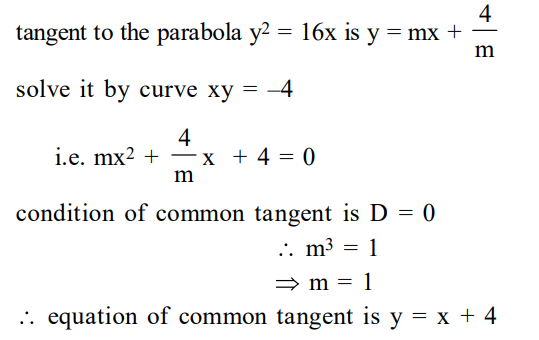# The equation of a common tangent to the curves,Question:

The equation of a common tangent to the curves, $y^{2}=16 x$ and $x y=-4$ is :

1. $x+y+4=0$

2. $x-2 y+16=0$

3. $2 x-y+2=0$

4. $x-y+4=0$

Correct Option: , 4

Solution: